# Train Generative Adversarial Network (GAN) for Sound Synthesis

This example shows how to train and use a generative adversarial network (GAN) to generate sounds.

### Introduction

In generative adversarial networks, a generator and a discriminator compete against each other to improve the generation quality.

GANs have generated significant interest in the field of audio and speech processing. Applications include text-to-speech synthesis, voice conversion, and speech enhancement.

This example trains a GAN for unsupervised synthesis of audio waveforms. The GAN in this example generates percussive sounds. The same approach can be followed to generate other types of sound, including speech.

### Synthesize Audio with Pre-Trained GAN

Before you train a GAN from scratch, use a pretrained GAN generator to synthesize percussive sounds.

```matFileName = "drumGeneratorWeights.mat"; loc = matlab.internal.examples.downloadSupportFile("audio","GanAudioSynthesis/" + matFileName); copyfile(loc,pwd)```

The function `synthesizePercussiveSound` calls a pretrained network to synthesize a percussive sound sampled at 16 kHz. The `synthesizePercussiveSound` function is included at the end of this example.

Synthesize a percussive sound and listen to it.

```synthsound = synthesizePercussiveSound; fs = 16e3; sound(synthsound,fs)```

Plot the synthesized percussive sound.

```t = (0:length(synthsound)-1)/fs; plot(t,synthsound) grid on xlabel("Time (s)") title("Synthesized Percussive Sound")```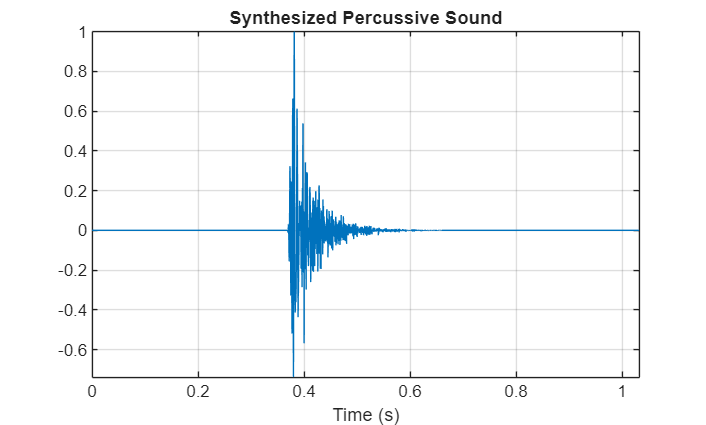You can use the percussive sounds synthesizer with other audio effects to create more complex applications. For example, you can apply reverberation to the synthesized percussive sounds.

Create a `reverberator` object and open its parameter tuner UI. This UI enables you to tune the `reverberator` parameters as the simulation runs.

```reverb = reverberator(SampleRate=fs); parameterTuner(reverb);```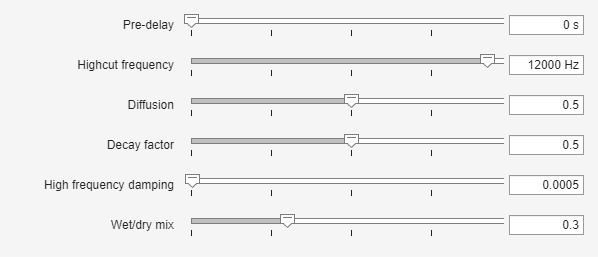Create a `timescope` object to visualize the percussive sounds.

```ts = timescope(SampleRate=fs, ... TimeSpanSource="Property", ... TimeSpanOverrunAction="Scroll", ... TimeSpan=10, ... BufferLength=10*256*64, ... ShowGrid=true, ... YLimits=[-1 1]);```

In a loop, synthesize the percussive sounds and apply reverberation. Use the parameter tuner UI to tune reverberation. If you want to run the simulation for a longer time, increase the value of the `loopCount` parameter.

```loopCount = 20; for ii = 1:loopCount synthsound = synthesizePercussiveSound; synthsound = reverb(synthsound); ts(synthsound(:,1)); soundsc(synthsound,fs) pause(0.5) end```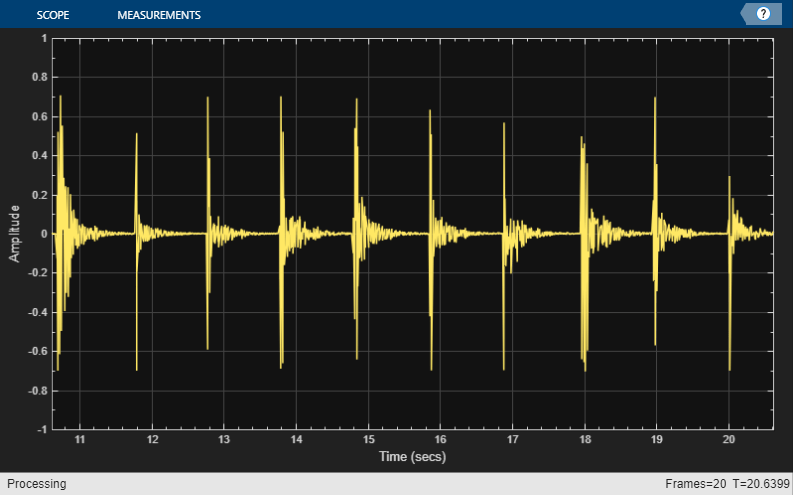### Train the GAN

Now that you have seen the pretrained percussive sounds generator in action, you can investigate the training process in detail.

A GAN is a type of deep learning network that generates data with characteristics similar to the training data.

A GAN consists of two networks that train together, a generator and a discriminator:

• Generator - Given a vector or random values as input, this network generates data with the same structure as the training data. It is the generator's job to fool the discriminator.

• Discriminator - Given batches of data containing observations from both the training data and the generated data, this network attempts to classify the observations as real or generated.

To maximize the performance of the generator, maximize the loss of the discriminator when given generated data. That is, the objective of the generator is to generate data that the discriminator classifies as real. To maximize the performance of the discriminator, minimize the loss of the discriminator when given batches of both real and generated data. Ideally, these strategies result in a generator that generates convincingly realistic data and a discriminator that has learned strong feature representations that are characteristic of the training data.

In this example, you train the generator to create fake time-frequency short-time Fourier transform (STFT) representations of percussive sounds. You train the discriminator to identify whether an STFT was synthesized by the generator or computed from a real audio signal. You create the real STFTs by computing the STFT of short recordings of real percussive sounds.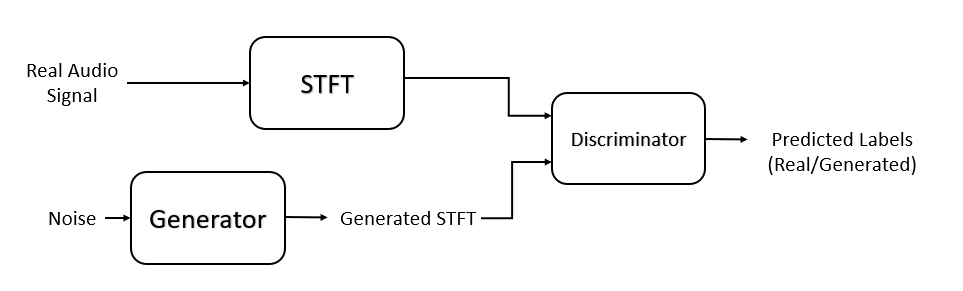Train a GAN using the Freesound One-Shot Percussive Sounds dataset . Download and extract the dataset. Remove any files with licenses that prohibit commercial use.

```url1 = "https://zenodo.org/record/4687854/files/one_shot_percussive_sounds.zip"; url2 = "https://zenodo.org/record/4687854/files/licenses.txt"; downloadFolder = tempdir; percussivesoundsFolder = fullfile(downloadFolder,"one_shot_percussive_sounds"); licensefilename = fullfile(percussivesoundsFolder,"licenses.txt"); if ~datasetExists(percussivesoundsFolder) disp("Downloading Freesound One-Shot Percussive Sounds Dataset (112.6 MB) ...") unzip(url1,downloadFolder) websave(licensefilename,url2); removeRestrictiveLicence(percussivesoundsFolder,licensefilename) end```

Create an `audioDatastore` object that points to the dataset.

`ads = audioDatastore(percussivesoundsFolder,IncludeSubfolders=true);`

### Define Generator Network

Define a network that generates STFTs from 1-by-1-by-100 arrays of random values. Create a network that upscales 1-by-1-by-100 arrays to 128-by-128-by-1 arrays using a fully connected layer followed by a reshape layer and a series of transposed convolution layers with ReLU layers.

This figure shows the dimensions of the signal as it travels through the generator. The generator architecture is defined in Table 4 of .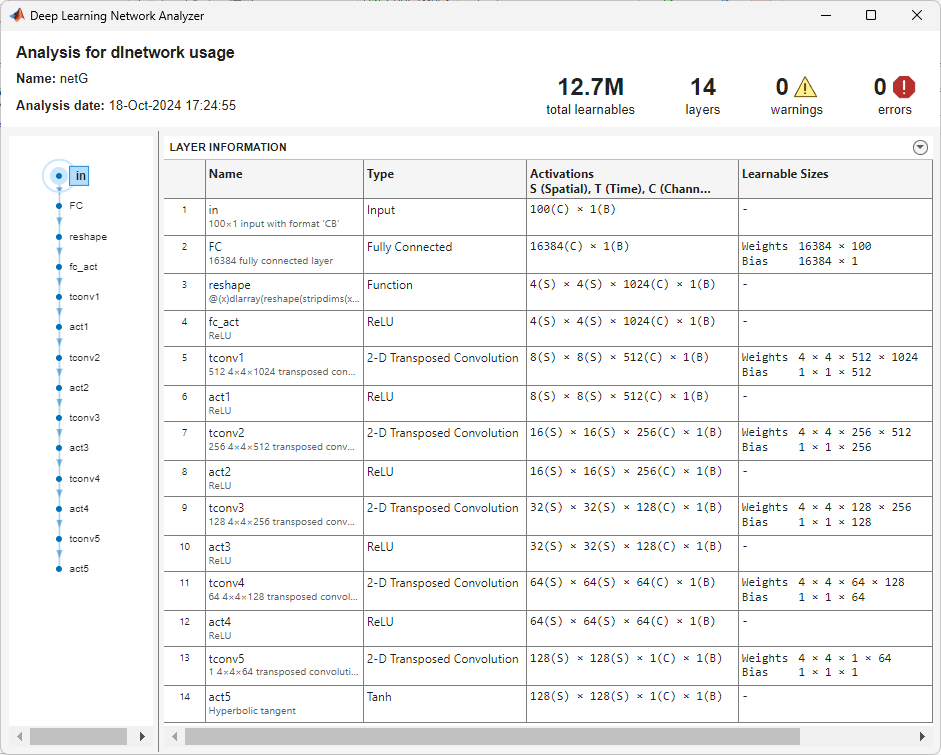The generator network is defined in `modelGenerator`, which is included at the end of this example.

### Define Discriminator Network

Define a network that classifies real and generated 128-by-128 STFTs.

Create a network that takes 128-by-128 images and outputs a scalar prediction score using a series of convolution layers with leaky ReLU layers followed by a fully connected layer.

This figure shows the dimensions of the signal as it travels through the discriminator. The discriminator architecture is defined in Table 5 of .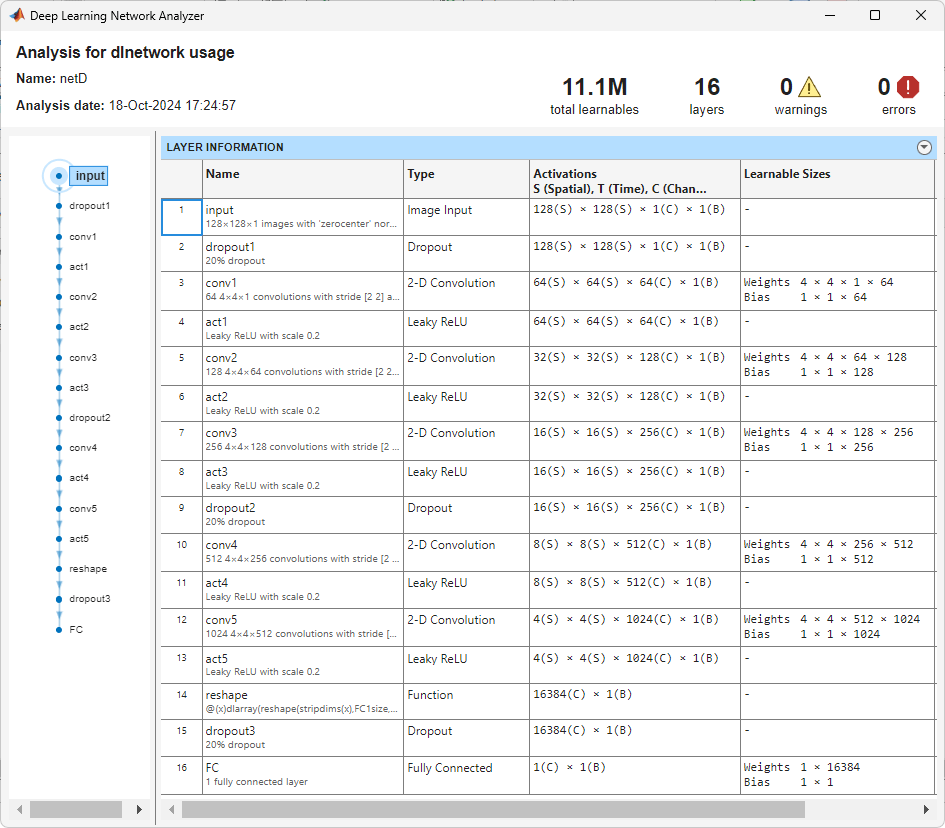The discriminator network is defined in `modelDiscriminator`, which is included at the end of this example.

### Generate Real Percussive Sounds Training Data

Generate STFT data from the percussive sound signals in the datastore.

Define the STFT parameters.

```fftLength = 256; win = hann(fftLength,"periodic"); overlapLength = 128;```

To speed up processing, distribute the feature extraction across multiple workers using `parfor`.

First, determine the number of partitions for the dataset. If you do not have Parallel Computing Toolbox™, use a single partition.

```if canUseParallelPool pool = gcp; numPar = numpartitions(ads,pool); else numPar = 1; end```
```Starting parallel pool (parpool) using the 'local' profile ... Connected to parallel pool with 6 workers. ```

For each partition, read from the datastore and compute the STFT.

```parfor ii = 1:numPar subds = partition(ads,numPar,ii); STrain = zeros(fftLength/2+1,128,1,numel(subds.Files)); for idx = 1:numel(subds.Files) % Read audio [x,xinfo] = read(subds); % Preprocess x = preprocessAudio(single(x),xinfo.SampleRate); % STFT S0 = stft(x,Window=win,OverlapLength=overlapLength,FrequencyRange="onesided"); % Magnitude S = abs(S0); STrain(:,:,:,idx) = S; end STrainC{ii} = STrain; end```
```Analyzing and transferring files to the workers ...done. ```

Convert the output to a four-dimensional array with STFTs along the fourth dimension.

`STrain = cat(4,STrainC{:});`

Convert the data to the log scale to better align with human perception.

`STrain = log(STrain + 1e-6);`

Normalize training data to have zero mean and unit standard deviation.

Compute the STFT mean and standard deviation of each frequency bin.

```SMean = mean(STrain,[2 3 4]); SStd = std(STrain,1,[2 3 4]);```

Normalize each frequency bin.

`STrain = (STrain-SMean)./SStd;`

The computed STFTs have unbounded values. Following the approach in , make the data bounded by clipping the spectra to 3 standard deviations and rescaling to [-1 1].

```STrain = STrain/3; Y = reshape(STrain,numel(STrain),1); Y(Y<-1) = -1; Y(Y>1) = 1; STrain = reshape(Y,size(STrain));```

Discard the last frequency bin to force the number of STFT bins to a power of two (which works well with convolutional layers).

`STrain = STrain(1:end-1,:,:,:);`

Permute the dimensions in preparation for feeding to the discriminator.

`STrain = permute(STrain,[2 1 3 4]);`

### Specify Training Options

Train with a mini-batch size of 64 for 1000 epochs.

```maxEpochs = 1000; miniBatchSize = 64;```

Compute the number of iterations required to consume the data.

`numIterationsPerEpoch = floor(size(STrain,4)/miniBatchSize);`

Specify the options for Adam optimization. Set the learn rate of the generator and discriminator to `0.0002`. For both networks, use a gradient decay factor of 0.5 and a squared gradient decay factor of 0.999.

```learnRateGenerator = 0.0002; learnRateDiscriminator = 0.0002; gradientDecayFactor = 0.5; squaredGradientDecayFactor = 0.999;```

Train on a GPU if one is available. Using a GPU requires Parallel Computing Toolbox™.

`executionEnvironment =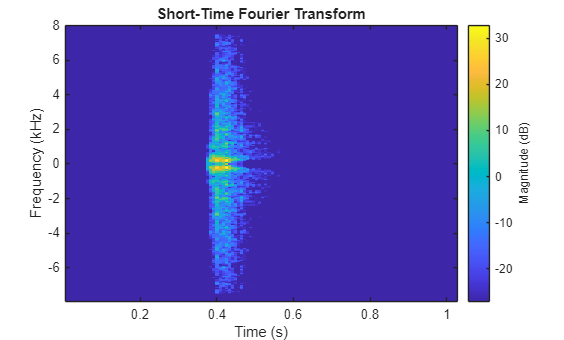"auto";`

Initialize the generator and discriminator weights. The `initializeGeneratorWeights` and `initializeDiscriminatorWeights` functions return random weights obtained using Glorot uniform initialization. The functions are included at the end of this example.

```generatorParameters = initializeGeneratorWeights; discriminatorParameters = initializeDiscriminatorWeights;```

### Train Model

Train the model using a custom training loop. Loop over the training data and update the network parameters at each iteration.

For each epoch, shuffle the training data and loop over mini-batches of data.

For each mini-batch:

• Generate a `dlarray` object containing an array of random values for the generator network.

• For GPU training, convert the data to a `gpuArray` (Parallel Computing Toolbox) object.

• Evaluate the model gradients using `dlfeval` (Deep Learning Toolbox) and the helper functions, `modelDiscriminatorGradients` and `modelGeneratorGradients`.

• Update the network parameters using the `adamupdate` (Deep Learning Toolbox) function.

```trailingAvgGenerator = []; trailingAvgSqGenerator = []; trailingAvgDiscriminator = []; trailingAvgSqDiscriminator = [];```

Depending on your machine, training this network can take hours. To skip training, set `doTraining` to `false`.

`doTraining =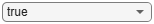true;`

You can set `saveCheckpoints` to `true` to save the updated weights and states to a MAT file every ten epochs. You can then use this MAT file to resume training if it is interrupted.

`saveCheckpoints =true;`

Specify the length of the generator input.

`numLatentInputs = 100;`

Train the GAN. This can take multiple hours to run.

```iteration = 0; for epoch = 1:maxEpochs % Shuffle the data. idx = randperm(size(STrain,4)); STrain = STrain(:,:,:,idx); % Loop over mini-batches. for index = 1:numIterationsPerEpoch iteration = iteration + 1; % Read mini-batch of data. dlX = STrain(:,:,:,(index-1)*miniBatchSize+1:index*miniBatchSize); dlX = dlarray(dlX,"SSCB"); % Generate latent inputs for the generator network. Z = 2 * ( rand(1,1,numLatentInputs,miniBatchSize,"single") - 0.5 ) ; dlZ = dlarray(Z); % If training on a GPU, then convert data to gpuArray. if (executionEnvironment == "auto" && canUseGPU) || executionEnvironment == "gpu" dlZ = gpuArray(dlZ); dlX = gpuArray(dlX); end % Evaluate the discriminator gradients using dlfeval and the % modelDiscriminatorGradients helper function. gradientsDiscriminator = ... dlfeval(@modelDiscriminatorGradients,discriminatorParameters,generatorParameters,dlX,dlZ); % Update the discriminator network parameters. [discriminatorParameters,trailingAvgDiscriminator,trailingAvgSqDiscriminator] = ... adamupdate(discriminatorParameters,gradientsDiscriminator, ... trailingAvgDiscriminator,trailingAvgSqDiscriminator,iteration, ... learnRateDiscriminator,gradientDecayFactor,squaredGradientDecayFactor); % Generate latent inputs for the generator network. Z = 2 * ( rand(1,1,numLatentInputs,miniBatchSize,"single") - 0.5 ) ; dlZ = dlarray(Z); % If training on a GPU, then convert data to gpuArray. if (executionEnvironment == "auto" && canUseGPU) || executionEnvironment == "gpu" dlZ = gpuArray(dlZ); end % Evaluate the generator gradients using dlfeval and the % |modelGeneratorGradients| helper function. gradientsGenerator = ... dlfeval(@modelGeneratorGradients,discriminatorParameters,generatorParameters,dlZ); % Update the generator network parameters. [generatorParameters,trailingAvgGenerator,trailingAvgSqGenerator] = ... adamupdate(generatorParameters,gradientsGenerator, ... trailingAvgGenerator,trailingAvgSqGenerator,iteration, ... learnRateGenerator,gradientDecayFactor,squaredGradientDecayFactor); end % Every 10 epochs, save a training snapshot to a MAT file. if mod(epoch,10)==0 disp("Epoch " + epoch + " out of " + maxEpochs + " complete."); if saveCheckpoints % Save checkpoint in case training is interrupted. save("audiogancheckpoint.mat", ... "generatorParameters","discriminatorParameters", ... "trailingAvgDiscriminator","trailingAvgSqDiscriminator", ... "trailingAvgGenerator","trailingAvgSqGenerator","iteration"); end end end```
```Epoch 10 out of 1000 complete. Epoch 20 out of 1000 complete. Epoch 30 out of 1000 complete. Epoch 40 out of 1000 complete. Epoch 50 out of 1000 complete. Epoch 60 out of 1000 complete. Epoch 70 out of 1000 complete. Epoch 80 out of 1000 complete. Epoch 90 out of 1000 complete. Epoch 100 out of 1000 complete. Epoch 110 out of 1000 complete. Epoch 120 out of 1000 complete. Epoch 130 out of 1000 complete. Epoch 140 out of 1000 complete. Epoch 150 out of 1000 complete. Epoch 160 out of 1000 complete. Epoch 170 out of 1000 complete. Epoch 180 out of 1000 complete. Epoch 190 out of 1000 complete. Epoch 200 out of 1000 complete. Epoch 210 out of 1000 complete. Epoch 220 out of 1000 complete. Epoch 230 out of 1000 complete. Epoch 240 out of 1000 complete. Epoch 250 out of 1000 complete. Epoch 260 out of 1000 complete. Epoch 270 out of 1000 complete. Epoch 280 out of 1000 complete. Epoch 290 out of 1000 complete. Epoch 300 out of 1000 complete. Epoch 310 out of 1000 complete. Epoch 320 out of 1000 complete. Epoch 330 out of 1000 complete. Epoch 340 out of 1000 complete. Epoch 350 out of 1000 complete. Epoch 360 out of 1000 complete. Epoch 370 out of 1000 complete. Epoch 380 out of 1000 complete. Epoch 390 out of 1000 complete. Epoch 400 out of 1000 complete. Epoch 410 out of 1000 complete. Epoch 420 out of 1000 complete. Epoch 430 out of 1000 complete. Epoch 440 out of 1000 complete. Epoch 450 out of 1000 complete. Epoch 460 out of 1000 complete. Epoch 470 out of 1000 complete. Epoch 480 out of 1000 complete. Epoch 490 out of 1000 complete. Epoch 500 out of 1000 complete. Epoch 510 out of 1000 complete. Epoch 520 out of 1000 complete. Epoch 530 out of 1000 complete. Epoch 540 out of 1000 complete. Epoch 550 out of 1000 complete. Epoch 560 out of 1000 complete. Epoch 570 out of 1000 complete. Epoch 580 out of 1000 complete. Epoch 590 out of 1000 complete. Epoch 600 out of 1000 complete. Epoch 610 out of 1000 complete. Epoch 620 out of 1000 complete. Epoch 630 out of 1000 complete. Epoch 640 out of 1000 complete. Epoch 650 out of 1000 complete. Epoch 660 out of 1000 complete. Epoch 670 out of 1000 complete. Epoch 680 out of 1000 complete. Epoch 690 out of 1000 complete. Epoch 700 out of 1000 complete. Epoch 710 out of 1000 complete. Epoch 720 out of 1000 complete. Epoch 730 out of 1000 complete. Epoch 740 out of 1000 complete. Epoch 750 out of 1000 complete. Epoch 760 out of 1000 complete. Epoch 770 out of 1000 complete. Epoch 780 out of 1000 complete. Epoch 790 out of 1000 complete. Epoch 800 out of 1000 complete. Epoch 810 out of 1000 complete. Epoch 820 out of 1000 complete. Epoch 830 out of 1000 complete. Epoch 840 out of 1000 complete. Epoch 850 out of 1000 complete. Epoch 860 out of 1000 complete. Epoch 870 out of 1000 complete. Epoch 880 out of 1000 complete. Epoch 890 out of 1000 complete. Epoch 900 out of 1000 complete. Epoch 910 out of 1000 complete. Epoch 920 out of 1000 complete. Epoch 930 out of 1000 complete. Epoch 940 out of 1000 complete. Epoch 950 out of 1000 complete. Epoch 960 out of 1000 complete. Epoch 970 out of 1000 complete. Epoch 980 out of 1000 complete. Epoch 990 out of 1000 complete. Epoch 1000 out of 1000 complete. ```

### Synthesize Sounds

Now that you have trained the network, you can investigate the synthesis process in more detail.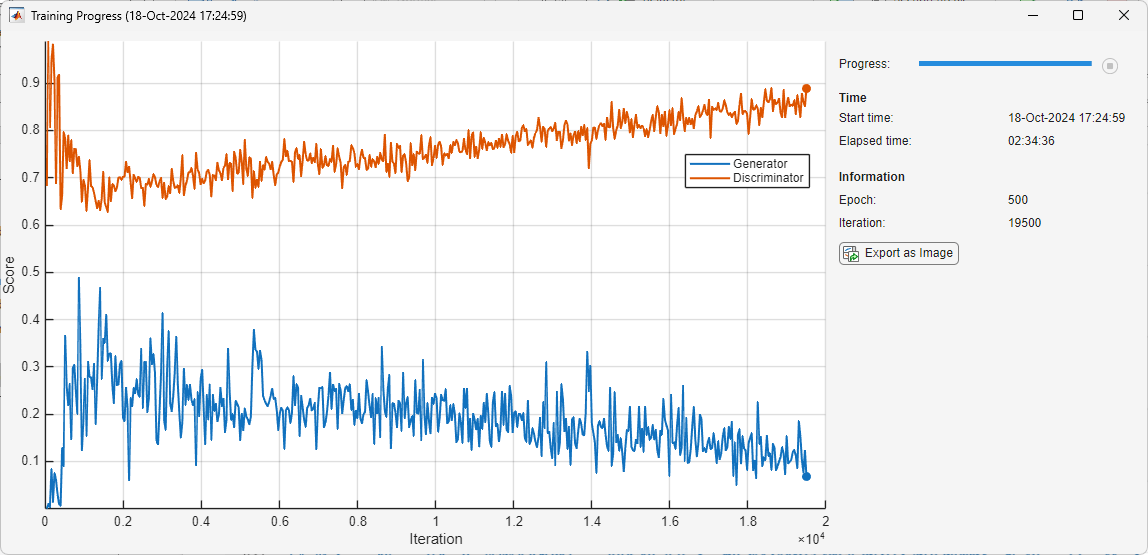The trained percussive sound generator synthesizes short-time Fourier transform (STFT) matrices from input arrays of random values. An inverse STFT (ISTFT) operation converts the time-frequency STFT to a synthesized time-domain audio signal.

If you skipped training, load the weights of a pretrained generator.

```if ~doTraining load(matFileName,"generatorParameters","SMean","SStd"); end```

The generator takes 1-by-1-by-100 vectors of random values as an input. Generate a sample input vector.

`dlZ = dlarray(2*(rand(1,1,numLatentInputs,1,"single") - 0.5));`

Pass the random vector to the generator to create an STFT image. `generatorParameters` is a structure containing the weights of the pretrained generator.

`dlXGenerated = modelGenerator(dlZ,generatorParameters);`

Convert the STFT `dlarray` to a single-precision matrix.

`S = dlXGenerated.extractdata;`

Transpose the STFT to align its dimensions with the `istft` function.

`S = S.';`

The STFT is a 128-by-128 matrix, where the first dimension represents 128 frequency bins linearly spaced from 0 to 8 kHz. The generator was trained to generate a one-sided STFT from an FFT length of 256, with the last bin omitted. Reintroduce that bin by inserting a row of zeros into the STFT.

`S = [S;zeros(1,128)];`

Revert the normalization and scaling steps used when you generated the STFTs for training.

```S = S * 3; S = (S.*SStd) + SMean;```

Convert the STFT from the log domain to the linear domain.

`S = exp(S);`

Convert the STFT from one-sided to two-sided.

`S = [S;S(end-1:-1:2,:)];`

Pad with zeros to remove window edge-effects.

`S = [zeros(256,100),S,zeros(256,100)];`

The STFT matrix does not contain any phase information. Use a fast version of the Griffin-Lim algorithm with 20 iterations to estimate the signal phase and produce audio samples.

```myAudio = stftmag2sig(S,256, ... FrequencyRange="twosided", ... Window=hann(256,"periodic"), ... OverlapLength=128, ... MaxIterations=20, ... Method="fgla"); myAudio = myAudio./max(abs(myAudio),[],"all"); myAudio = myAudio(128*100:end-128*100);```

Listen to the synthesized percussive sound.

`sound(gather(myAudio),fs)`

Plot the synthesized percussive sound.

```t = (0:length(myAudio)-1)/fs; plot(t,myAudio) grid on xlabel("Time (s)") title("Synthesized GAN Sound")```Plot the STFT of the synthesized percussive sound.

```figure stft(myAudio,fs,Window=hann(256,"periodic"),OverlapLength=128);```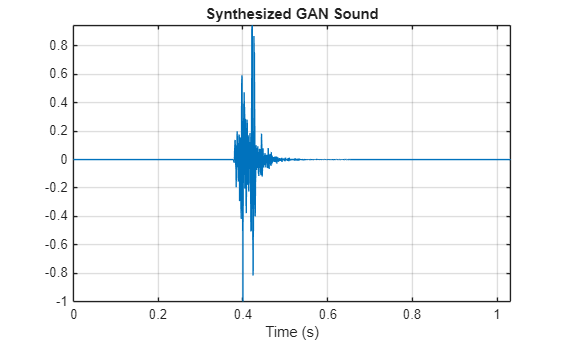### Model Generator Function

The `modelGenerator` function upscales 1-by-1-by-100 arrays (dlX) to 128-by-128-by-1 arrays (dlY). `parameters` is a structure holding the weights of the generator layers. The generator architecture is defined in Table 4 of .

```function dlY = modelGenerator(dlX,parameters) dlY = fullyconnect(dlX,parameters.FC.Weights,parameters.FC.Bias,Dataformat="SSCB"); dlY = reshape(dlY,[1024 4 4 size(dlY,2)]); dlY = permute(dlY,[3 2 1 4]); dlY = relu(dlY); dlY = dltranspconv(dlY,parameters.Conv1.Weights,parameters.Conv1.Bias,Stride=2,Cropping="same",DataFormat="SSCB"); dlY = relu(dlY); dlY = dltranspconv(dlY,parameters.Conv2.Weights,parameters.Conv2.Bias,Stride=2,Cropping="same",DataFormat="SSCB"); dlY = relu(dlY); dlY = dltranspconv(dlY,parameters.Conv3.Weights,parameters.Conv3.Bias,Stride=2,Cropping="same",DataFormat="SSCB"); dlY = relu(dlY); dlY = dltranspconv(dlY,parameters.Conv4.Weights,parameters.Conv4.Bias,Stride=2,Cropping="same",DataFormat="SSCB"); dlY = relu(dlY); dlY = dltranspconv(dlY,parameters.Conv5.Weights,parameters.Conv5.Bias,Stride=2,Cropping="same",DataFormat="SSCB"); dlY = tanh(dlY); end```

### Model Discriminator Function

The `modelDiscriminator` function takes 128-by-128 images and outputs a scalar prediction score. The discriminator architecture is defined in Table 5 of .

```function dlY = modelDiscriminator(dlX,parameters) dlY = dlconv(dlX,parameters.Conv1.Weights,parameters.Conv1.Bias,Stride=2,Padding="same"); dlY = leakyrelu(dlY,0.2); dlY = dlconv(dlY,parameters.Conv2.Weights,parameters.Conv2.Bias,Stride=2,Padding="same"); dlY = leakyrelu(dlY,0.2); dlY = dlconv(dlY,parameters.Conv3.Weights,parameters.Conv3.Bias,Stride=2,Padding="same"); dlY = leakyrelu(dlY,0.2); dlY = dlconv(dlY,parameters.Conv4.Weights,parameters.Conv4.Bias,Stride=2,Padding="same"); dlY = leakyrelu(dlY,0.2); dlY = dlconv(dlY,parameters.Conv5.Weights,parameters.Conv5.Bias,Stride=2,Padding="same"); dlY = leakyrelu(dlY,0.2); dlY = stripdims(dlY); dlY = permute(dlY,[3 2 1 4]); dlY = reshape(dlY,4*4*64*16,numel(dlY)/(4*4*64*16)); weights = parameters.FC.Weights; bias = parameters.FC.Bias; dlY = fullyconnect(dlY,weights,bias,Dataformat="CB"); end```

The `modelDiscriminatorGradients` functions takes as input the generator and discriminator parameters `generatorParameters` and `discriminatorParameters`, a mini-batch of input data `X`, and an array of random values `Z`, and returns the gradients of the discriminator loss with respect to the learnable parameters in the networks.

```function gradientsDiscriminator = modelDiscriminatorGradients(discriminatorParameters,generatorParameters,X,Z) % Calculate the predictions for real data with the discriminator network. Y = modelDiscriminator(X,discriminatorParameters); % Calculate the predictions for generated data with the discriminator network. Xgen = modelGenerator(Z,generatorParameters); Ygen = modelDiscriminator(dlarray(Xgen,"SSCB"),discriminatorParameters); % Calculate the GAN loss. lossDiscriminator = ganDiscriminatorLoss(Y,Ygen); % For each network, calculate the gradients with respect to the loss. gradientsDiscriminator = dlgradient(lossDiscriminator,discriminatorParameters); end```

The `modelGeneratorGradients` function takes as input the discriminator and generator learnable parameters and an array of random values `Z`, and returns the gradients of the generator loss with respect to the learnable parameters in the networks.

```function gradientsGenerator = modelGeneratorGradients(discriminatorParameters,generatorParameters,Z) % Calculate the predictions for generated data with the discriminator network. Xgen = modelGenerator(Z,generatorParameters); Ygen = modelDiscriminator(dlarray(Xgen,"SSCB"),discriminatorParameters); % Calculate the GAN loss lossGenerator = ganGeneratorLoss(Ygen); % For each network, calculate the gradients with respect to the loss. gradientsGenerator = dlgradient(lossGenerator,generatorParameters); end```

### Discriminator Loss Function

The objective of the discriminator is to not be fooled by the generator. To maximize the probability that the discriminator successfully discriminates between the real and generated images, minimize the discriminator loss function. The loss function for the generator follows the DCGAN approach highlighted in .

```function lossDiscriminator = ganDiscriminatorLoss(dlYPred,dlYPredGenerated) fake = dlarray(zeros(1,size(dlYPred,2))); real = dlarray(ones(1,size(dlYPred,2))); D_loss = mean(sigmoid_cross_entropy_with_logits(dlYPredGenerated,fake)); D_loss = D_loss + mean(sigmoid_cross_entropy_with_logits(dlYPred,real)); lossDiscriminator = D_loss / 2; end```

### Generator Loss Function

The objective of the generator is to generate data that the discriminator classifies as "real". To maximize the probability that images from the generator are classified as real by the discriminator, minimize the generator loss function. The loss function for the generator follows the deep convolutional generative adverarial network (DCGAN) approach highlighted in .

```function lossGenerator = ganGeneratorLoss(dlYPredGenerated) real = dlarray(ones(1,size(dlYPredGenerated,2))); lossGenerator = mean(sigmoid_cross_entropy_with_logits(dlYPredGenerated,real)); end```

### Discriminator Weights Initializer

`initializeDiscriminatorWeights` initializes discriminator weights using the Glorot algorithm.

```function discriminatorParameters = initializeDiscriminatorWeights filterSize = [5 5]; dim = 64; % Conv2D weights = iGlorotInitialize([filterSize(1) filterSize(2) 1 dim]); bias = zeros(1,1,dim,"single"); discriminatorParameters.Conv1.Weights = dlarray(weights); discriminatorParameters.Conv1.Bias = dlarray(bias); % Conv2D weights = iGlorotInitialize([filterSize(1) filterSize(2) dim 2*dim]); bias = zeros(1,1,2*dim,"single"); discriminatorParameters.Conv2.Weights = dlarray(weights); discriminatorParameters.Conv2.Bias = dlarray(bias); % Conv2D weights = iGlorotInitialize([filterSize(1) filterSize(2) 2*dim 4*dim]); bias = zeros(1,1,4*dim,"single"); discriminatorParameters.Conv3.Weights = dlarray(weights); discriminatorParameters.Conv3.Bias = dlarray(bias); % Conv2D weights = iGlorotInitialize([filterSize(1) filterSize(2) 4*dim 8*dim]); bias = zeros(1,1,8*dim,"single"); discriminatorParameters.Conv4.Weights = dlarray(weights); discriminatorParameters.Conv4.Bias = dlarray(bias); % Conv2D weights = iGlorotInitialize([filterSize(1) filterSize(2) 8*dim 16*dim]); bias = zeros(1,1,16*dim,"single"); discriminatorParameters.Conv5.Weights = dlarray(weights); discriminatorParameters.Conv5.Bias = dlarray(bias); % fully connected weights = iGlorotInitialize([1,4 * 4 * dim * 16]); bias = zeros(1,1,"single"); discriminatorParameters.FC.Weights = dlarray(weights); discriminatorParameters.FC.Bias = dlarray(bias); end```

### Generator Weights Initializer

`initializeGeneratorWeights` initializes generator weights using the Glorot algorithm.

```function generatorParameters = initializeGeneratorWeights dim = 64; % Dense 1 weights = iGlorotInitialize([dim*256,100]); bias = zeros(dim*256,1,"single"); generatorParameters.FC.Weights = dlarray(weights); generatorParameters.FC.Bias = dlarray(bias); filterSize = [5 5]; % Trans Conv2D weights = iGlorotInitialize([filterSize(1) filterSize(2) 8*dim 16*dim]); bias = zeros(1,1,dim*8,"single"); generatorParameters.Conv1.Weights = dlarray(weights); generatorParameters.Conv1.Bias = dlarray(bias); % Trans Conv2D weights = iGlorotInitialize([filterSize(1) filterSize(2) 4*dim 8*dim]); bias = zeros(1,1,dim*4,"single"); generatorParameters.Conv2.Weights = dlarray(weights); generatorParameters.Conv2.Bias = dlarray(bias); % Trans Conv2D weights = iGlorotInitialize([filterSize(1) filterSize(2) 2*dim 4*dim]); bias = zeros(1,1,dim*2,"single"); generatorParameters.Conv3.Weights = dlarray(weights); generatorParameters.Conv3.Bias = dlarray(bias); % Trans Conv2D weights = iGlorotInitialize([filterSize(1) filterSize(2) dim 2*dim]); bias = zeros(1,1,dim,"single"); generatorParameters.Conv4.Weights = dlarray(weights); generatorParameters.Conv4.Bias = dlarray(bias); % Trans Conv2D weights = iGlorotInitialize([filterSize(1) filterSize(2) 1 dim]); bias = zeros(1,1,1,"single"); generatorParameters.Conv5.Weights = dlarray(weights); generatorParameters.Conv5.Bias = dlarray(bias); end```

### Synthesize Percussive Sound

`synthesizePercussiveSound` uses a pretrained network to synthesize percussive sounds.

```function y = synthesizePercussiveSound persistent pGeneratorParameters pMean pSTD if isempty(pGeneratorParameters) % If the MAT file does not exist, download it filename = "drumGeneratorWeights.mat"; load(filename,"SMean","SStd","generatorParameters"); pMean = SMean; pSTD = SStd; pGeneratorParameters = generatorParameters; end % Generate random vector dlZ = dlarray(2 * ( rand(1,1,100,1,"single") - 0.5 )); % Generate spectrograms dlXGenerated = modelGenerator(dlZ,pGeneratorParameters); % Convert from dlarray to single S = dlXGenerated.extractdata; S = S.'; % Zero-pad to remove edge effects S = [S ; zeros(1,128)]; % Reverse steps from training S = S * 3; S = (S.*pSTD) + pMean; S = exp(S); % Make it two-sided S = [S ; S(end-1:-1:2,:)]; % Pad with zeros at end and start S = [zeros(256,100) S zeros(256,100)]; % Reconstruct the signal using a fast Griffin-Lim algorithm. myAudio = stftmag2sig(S,256, ... FrequencyRange="twosided", ... Window=hann(256,"periodic"), ... OverlapLength=128, ... MaxIterations=20, ... Method="fgla"); myAudio = myAudio./max(abs(myAudio),[],"all"); y = myAudio(128*100:end-128*100); end```

### Utility Functions

```function out = sigmoid_cross_entropy_with_logits(x,z) out = max(x, 0) - x .* z + log(1 + exp(-abs(x))); end function w = iGlorotInitialize(sz) if numel(sz) == 2 numInputs = sz(2); numOutputs = sz(1); else numInputs = prod(sz(1:3)); numOutputs = prod(sz([1 2 4])); end multiplier = sqrt(2 / (numInputs + numOutputs)); w = multiplier * sqrt(3) * (2 * rand(sz,"single") - 1); end function out = preprocessAudio(in,fs) % Ensure mono in = mean(in,2); % Resample to 16kHz x = resample(in,16e3,fs); % Cut or pad to have approximately 1-second length plus padding to ensure % 128 analysis windows for an STFT with 256-point window and 128-point % overlap. y = trimOrPad(x,16513); % Normalize out = y./max(abs(y)); end function y = trimOrPad(x,n) %trimOrPad Trim or pad audio % % y = trimOrPad(x,n) trims or pads the input x to n samples along the first % dimension. If x is trimmed, it is trimmed equally on the front and back. % If x is padded, it is padded equally on the front and back with zeros. % For odd-length trimming or padding, the extra sample is trimmed or padded % from the back. a = size(x,1); if a < n frontPad = floor((n-a)/2); backPad = n - a - frontPad; y = [zeros(frontPad,size(x,2),like=x);x;zeros(backPad,size(x,2),like=x)]; elseif a > n frontTrim = floor((a-n)/2) + 1; backTrim = a - n - frontTrim; y = x(frontTrim:end-backTrim,:); else y = x; end end function removeRestrictiveLicence(percussivesoundsFolder,licensefilename) %removeRestrictiveLicense Remove restrictive license % Parse the licenses file that maps ids to license. Create a table to hold the info. f = fileread(licensefilename); K = jsondecode(f); fns = fields(K); T = table(Size=[numel(fns),4], ... VariableTypes=["string","string","string","string"], ... VariableNames=["ID","FileName","UserName","License"]); for ii = 1:numel(fns) fn = string(K.(fns{ii}).name); li = string(K.(fns{ii}).license); id = extractAfter(string(fns{ii}),"x"); un = string(K.(fns{ii}).username); T(ii,:) = {id,fn,un,li}; end % Remove any files that prohibit commercial use. Find the file inside the % appropriate folder, and then delete it. unsupportedLicense = "http://creativecommons.org/licenses/by-nc/3.0/"; fileToRemove = T.ID(strcmp(T.License,unsupportedLicense)); for ii = 1:numel(fileToRemove) fileInfo = dir(fullfile(percussivesoundsFolder,"**",fileToRemove(ii)+".wav")); delete(fullfile(fileInfo.folder,fileInfo.name)) end end```

### Reference

 Donahue, C., J. McAuley, and M. Puckette. 2019. "Adversarial Audio Synthesis." ICLR.

 Ramires, Antonio, Pritish Chandna, Xavier Favory, Emilia Gomez, and Xavier Serra. "Neural Percussive Synthesis Parameterised by High-Level Timbral Features." ICASSP 2020 - 2020 IEEE International Conference on Acoustics, Speech and Signal Processing (ICASSP), 2020. https://doi.org/10.1109/icassp40776.2020.9053128.